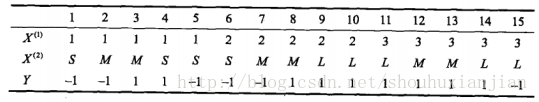# 贝叶斯-笔记(1)

### 0 - 背景

$P(X,Y)=P(X|Y)P(Y) \tag{0.1}$

### 1 - 朴素贝叶斯

所谓朴素贝叶斯，就是基于特征条件是相互独立的假设下，学习输入和输出的联合概率分布，所以它是一个生成模型。
假设输入空间是$\chi \subseteq R^n$为n维向量的集合，输出空间是类别标记集合（标签）$y \in \cal Y$.X是定义在输入空间$\chi$上的随机向量，Y是定义在输出空间$\cal Y$上的随机变量。P(X,Y)是X和Y的联合概率分布。假设训练集:

$T={(x_1,y_1),(x_2,y_2),...,(x_N,y_N)}$

$P(X=x|Y=c_k)=P(X^{(1)},X^{(2)},...,X^{(n)}|Y=c_k)$

$\begin{eqnarray} P(X=x|Y=c_k) &=&P(X^{(1)}=x^{(1)},X^{(n)}=x^{(n)}|Y=c_k)\\ &=&\prod_{i=1}^nP(X^{(i)}=x^{(i)}|Y=c_k) \end{eqnarray}$

$\begin{eqnarray}P(Y=c_k|X=x) &=&\frac{\frac{P(Y=c_k,X=x)}{P(X=x)}}{\frac{\sum_i^KP(Y=c_i,X=x)}{P(X=x)}}\\ &=& \frac{P(Y=c_k,X=x)}{\sum_i^KP(Y=c_i,X=x)}\\ &=& \frac{P(X=x|Y=c_k)P(Y=c_k)}{\sum_i^KP(X=x|Y=c_i)P(Y=c_i)}\\ &=& \frac{P(Y=c_k)\prod_{i=1}^nP(X^{(i)}=x^{(i)}|Y=c_k)}{\sum_i^KP(Y=c_k)\prod_{i=1}^nP(X^{(i)}=x^{(i)}|Y=c_k)} \end{eqnarray}$

$y=f(x)=argmax_{c_k}P(Y=c_k|X=x)$

$y=argmax_{c_k}P(Y=c_k)\prod_{i=1}^nP(X^{(i)}=x^{(i)}|Y=c_k)$

$L(Y,f(X))= \begin{cases} 1,&\text Y\neq f(X) \\ 0,& \text Y=f(X) \end{cases}$

$R_exp(f)=E[L(Y,f(X))]$

ps:《概率论及其应用 第一卷第三版》170页：

$E(X)=\sum x_kf(x_k)$

$E(\phi(X))=\sum\phi(x_k)f(x_k)$

$R_exp(f) = \sum_{k=1}^KL(c_k,f(x))P(c_k|x)$

$\begin{eqnarray}f(x) &=&{argmin}_{y \in \cal Y}\sum_{k=1}^KL(c_k,y)P(c_k|x)\\ &=&argmin_{y \in \cal Y}\sum_{k=1}^KP(y \neq c_k|x)\\ &=&argmin_{y \in \cal Y}(1-P(y=c_k|x))\\ &=&argmax_{y \in \cal Y}P(y=c_k|x)\\ &=&argmax_{c_k}P(c_k|x) \end{eqnarray}$

#### 1.1 朴素贝叶斯学习过程：$P(Y=1)=\frac{9}{15},P(Y=-1)=\frac{6}{15}$

$P(X^{(1)}=1|Y=1)=\frac{2}{9}$,$P(X^{(1)}=2|Y=1)=\frac{3}{9}$,$P(X^{(1)}=3|Y=1)=\frac{4}{9}$
$P(X^{(2)}=S|Y=1)=\frac{1}{9}$,$P(X^{(2)}=M|Y=1)=\frac{4}{9}$,$P(X^{(2)}=L|Y=1)=\frac{4}{9}$

$P(X^{(1)}=1|Y=-1)=\frac{3}{6}$,$P(X^{(1)}=2|Y=-1)=\frac{2}{6}$,$P(X^{(1)}=3|Y=-1)=\frac{1}{6}$
$P(X^{(2)}=S|Y=-1)=\frac{3}{6}$,$P(X^{(2)}=M|Y=-1)=\frac{2}{6}$,$P(X^{(2)}=L|Y=-1)=\frac{1}{6}$

$P(Y=1)P(X^{(1)}=2|Y=1)P(X^{(2)}=S|Y=1)=\frac{9}{15}\frac{3}{9}\frac{1}{9}=\frac{1}{45}$

$P(Y=-1)P(X^{(1)}=2|Y=-1)P(X^{(2)}=S|Y=-1)=\frac{6}{15}\frac{2}{6}\frac{3}{6}=\frac{1}{15}$

1）条件概率的贝叶斯估计是：

$P_\lambda(X^{(j)}=a_{jl}|Y=c_k)=\frac{\sum_{i=1}^N I(x_i^{(j)}=a_{jl},y_i=c_k)+\lambda}{\sum_{i=1}^N I(y_i=c_k)+S_j\lambda}$

$P_\lambda(Y=c_k)=\frac{\sum_{i=1}^N I(y_i=c_k)+\lambda}{N+K\lambda}$

2017/03/27 第一次修改！

[] 李航，统计学习方法

posted @ 2018-10-11 16:13  仙守  阅读(470)  评论(0编辑  收藏  举报